Mobius strip

Definition

The Mobius strip, or twisted cylinder, is defined as follows.

• There is a midcircle of radius$R$
• There is a half-width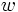$w$

Then the Mobius strip is the trace of a moving open line segment of length twice the half-width whose center traces the midcircle, and which rotates at a rate of half that at which it revolves.

There is also another, diffeomorphic, notion of Mobius strip which is very different in the sense of its metric. Check out flat Mobius strip.

Equational descriptions

Cartesian parametric equation

The Cartesian equation is given as follows, where$t$ varies in$[0,2\pi)$ and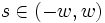$s \in (-w,w)$:

•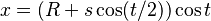$x = (R + s \cos (t/2)) \cos t$
•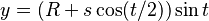$y = (R + s \cos (t/2)) \sin t$
•$z = R \sin (t/2)$

Abstract structure

Topological structure

Topologically, the Mobius strip can be viewed as: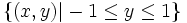$\{ (x,y) | -1 \le y \le 1 \}$

modulo the equivalence relation: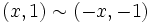$(x,1) \sim (-x,-1)$

Related surfaces

The Mobius strip can be identified with the set of all lines in the affine plane, and hence, its one-point compatification is that set plus the line at infinity, which is the projective plane.

One approach to compactifying the Mobius strip is to identify the two bounding sides, which, locally, looks like identifying the endpoints of each line segment. This gives rise to the Klein bottle.

The Klein bottle, however, cannot be embedded in$\R^3$. It can be described topologically as follws: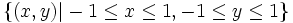$\{ (x,y) | -1 \le x \le 1, -1 \le y \le 1 \}$

modulo the following equivalence relations:$(x,1) \sim (-x,-1), (1,y) \sim (-1,y)$

The Mobius strip has a double cover which is the right circular cylinder. However, the pull-back metric on this is not the same as the usual metric on the right circular cylinder.

Structure and symmetry

Homeomorphisms

Topologically, any two points on the Mobius strip are equivalent, viz there is a homeomorphism taking any point to any other point.

Isometries

The Mobius strip seems to have no nontrivial isometry.

Properties

The Mobius strip is a nonorientable surface. Thus, any compactification of it is also nonorientable. Hence, from the fact that for compact surfaces orientability is equivalent to embeddability in$\R^3$, we conclude that no compactification of the Mobius strip can be embedded in$\R^3$.

From the curvature computations above, it is clear that the Gaussian curvature of the Mobius strip is everywhere negative.

At every point on the Mobius strip, it is possible to draw a lien segment containing that point that lies entirely on the Mobius strip.